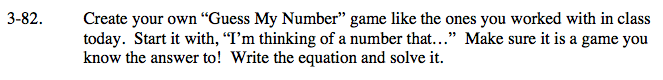### Home > CAAC > Chapter 3 > Lesson 3.2.2 > Problem3-82

3-82.I'm thinking of a number that when I double my number, add 2, subtract my number, and add 5, I get double my number.

Write an equation for the word problem.

2x + 2 − x + 5 = 2x

Simplify.

x + 7 = 2x

7 = x

Now create your own Guess My Number problem.